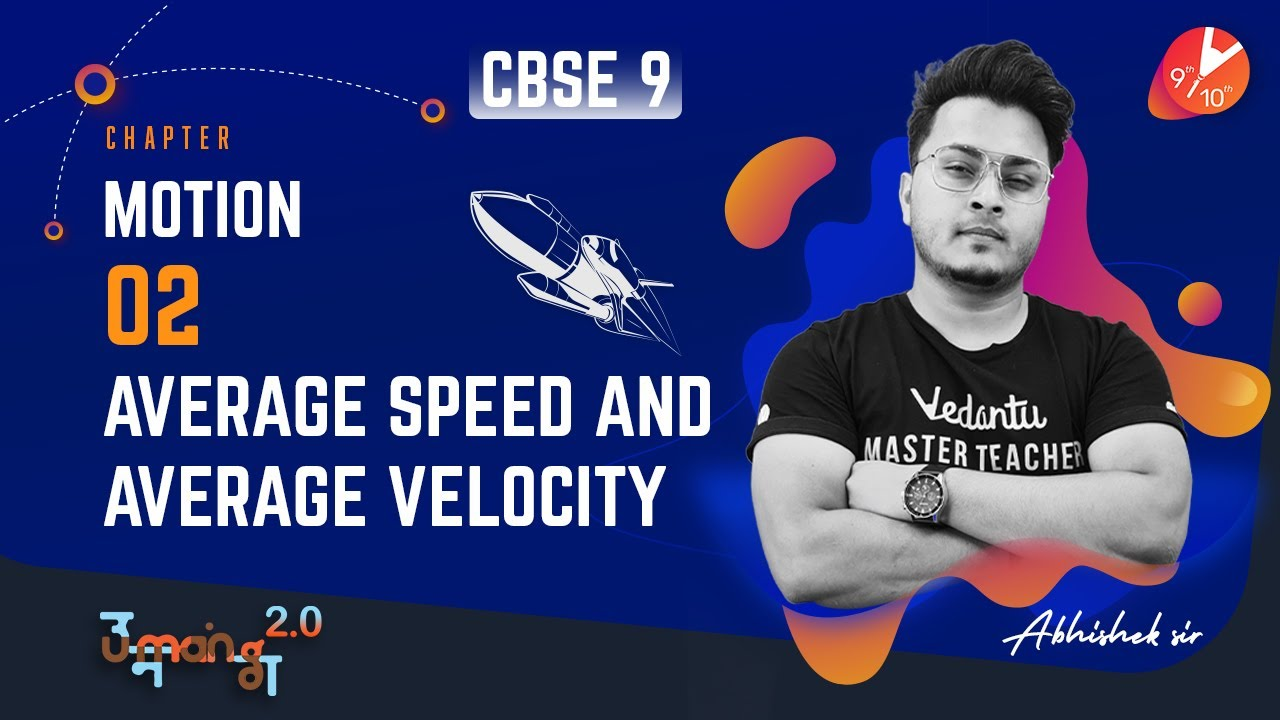## Understand the Difference Between Average Speed and Average Velocity

By: marysmith

We cannot perceive the difference between average speed and average velocity without learning average speed vs average velocity.

• Distance – it is a scalar quantity that tells how much path is covered by an object. Distance = speed x time
• Displacement is the smallest distance possible between the beginning point and ending point; displacement is a vector quantity.

For example: if a person is walking in a park with a circular track, after completing a single round, the distance will be the total path covered which is the perimeter, and the displacement will be zero because the starting point of walk and ending point of the walk is same.

• Speed – speed has no direction; it tells how much fast an object is moving. It is a scalar quantity. If an object is moving fast, it means it has fast speed, and if an object is moving slowly, it means it has slow speed. Speed is based on the distance travelled by an object, and it does not involve direction.

Speed = distance / time

• Velocity – it gives the rate at which an object is changing its position. It is a vector quantity which means it has both direction and magnitude. We can easily find the direction of the velocity vector.

It has the same direction as a moving object, and it does not depend on speed which means if an object is slowing down, the direction would still be the same. Velocity is based on the displacement of an object, and it does involve direction.

## What Is Average Speed?

Average is the total distance covered by an object divided by the total time taken to cover the said distance. It provides you with an average rate at which an object can cover a specified distance in a specified time.

To understand this better, let us take a situation;

Suppose Ram is driving his car at the speed of 60km/hr, which means that Ram will change its position from 60 km in every hour of his drive.

Formula to calculate Average speed = total distance / total time

## Examples

Shyam is driving his car; he covers 90 km in 3 hours. What is his average speed?

Solution- Total distance covered = 90 km

Total time taken = 3 hours

Average speed=total distance /total time

= 90km/3hours

= 30km/hrKamal is travelling from Delhi to Agra. He drove his car at 40km/hr for 4 hours and 90km/ for 3 hours, calculate his average speed?

Solution- distance = speed x time

For driving 4 hours, distance covered = 40 x 4 = 160km

For driving 3 hours, distance covered = 90 x 3 = 270km

Total distance covered by Kamal = 430km

Total time taken by Kamal = 7 hours

Average speed = total distance / total time

=  430/7 = 61.4 km/hr

## What Is Average Velocity?

The average velocity is provided by the total displacement of the object in a specified time divided by the time taken. The direction of average velocity is the same as the direction of the object; it doesn’t matter whether the speed is increasing or decreasing.

The direction will remain the same as the direction of displacement.

The formula of average velocity =total displacement / total time

## Examples

Rohan drives his car towards 60 km north in 2 hours and then takes a U-turn and drives toward 20km south in 1 hour. Calculate the total velocity of Rohan?

Solution;

Total displacement = 60 – 20 =40

Total time = 1 2=3

Average velocity=total displacement/ total time

= 40/3= 13.33 km/hr

A plane is flying 400 km towards the east in 3 hours and then take a turn and fly 150 km west in 2 hours. Find the average velocity?

solution : total displacement = 400 – 150= 250 km

total time =3 – 2= 1 hour

average velocity = total displacement/total time

250/1 =250 km/hr

Difference between average velocity and average speed

• Average speed depends on the distance covered by a particular object on a particular path. It is a scalar quantity while average velocity depends on the total displacement of an object, and the direction will remain the same.
• Average speed is always positive. The reason behind this is the total distance and total time can not be negative
• Average velocity can be negative as well as positive. The sign tells the direction of a moving object
• The average speed and average velocity will be equal if the object is moving in a straight line without changing its direction
• If an object changes direction, then the average speed will be greater than the average velocity.

Conclusion

So after understanding the average speed and average velocity, we concluded that average speed depends on distance and average velocity depends on displacement.